# Defining a Damping Set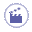This task will show you how to define the damping set in a Dynamic Response Analysis case.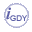Only available with the Generative Dynamic Response Analysis (GDY) product.Defining a damping set allows you to define the resulting damping of the part once the force has been applied to this part.
You can choose between two damping types: Modal or Rayleigh.
By default, the damping is modal.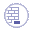Open the sample57.CATAnalysis document from the samples directory.

In this particular example, a dynamic response case and a modulation set have been already inserted.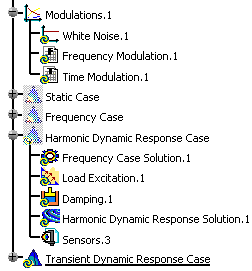1. Double-click the damping set from the specification tree.

 In this particular case, double-click the Damping.1 object. The Damping Choice dialog box appears.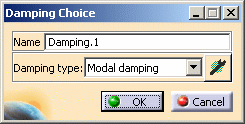Name: if needed, you can change the name of the damping set. Damping type:
2. Select the desired Damping type.#### Modal Damping Type

The modal damping is a fraction of the critical damping.
The critical damping is computed as follow: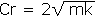where m is the mass of the system and k the stiffness of the system.#### Rayleigh Damping Type

The Rayleigh damping is defined as follow: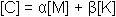where [M] is the mass matrix, [K] is the stiffness matrix.

3. Compute the frequency solution.

 For more details, refer to Computing Frequency Solutions.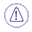You have to compute the frequency solution before defining the damping parameters.
4. Click the Component edition button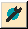to define the damping parameters.

The Damping Definition dialog box appears.

#### Modal Damping Definition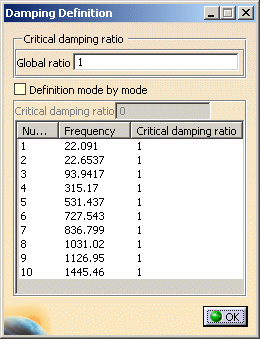• Global ratio: lets you define the factor of the critical damping for all the modes (in %).
• Definition mode by mode: lets you define the critical damping ratio (in %) independently for each mode.
Multi-selection is available in this case.

#### Rayleigh Damping Definition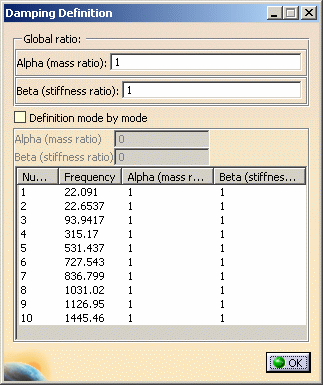• Global ratio: lets you define the Alpha (mass ratio) and/or Beta (stiffness ratio) coefficients for all the modes.
• Alpha (mass ratio): lets you define the factor of the mass ratio (in %).
• Beta (stiffness ratio): lets you define the factor of the stiffness ratio (in %).
• Definition mode by mode: lets you define the Alpha (mass ratio) and/or Beta (stiffness ratio) coefficients (in %) independently for each selected mode.
Multi-selection is available in this case.
5. Define the desired damping parameters and click OK in the Damping Choice dialog box.

6. Click OK in the Damping Definition dialog box.